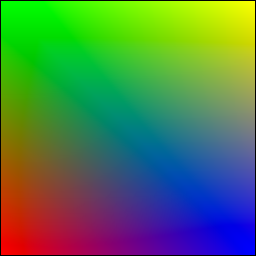# The easy way¶

As we’ve seen in the previous section, displaying a simple quad using modern GL is quite tedious and requires a fair number of operations. The goal of glumpy is to make this process both easy and intuitive. Let’s consider again the vertex and fragment code:

```vertex = """
uniform float scale;
attribute vec2 position;
attribute vec4 color;
varying vec4 v_color;
void main()
{
gl_Position = vec4(scale*position, 0.0, 1.0);
v_color = color;
} """

fragment = """
varying vec4 v_color;
void main()
{
gl_FragColor = v_color;
} """
```

The exact same example can now be rewritten as:

```from glumpy import app, gloo, gl

# Build the program and corresponding buffers (with 4 vertices)
quad = gloo.Program(vertex, fragment, count=4)

# Upload data into GPU
quad['color'] = [ (1,0,0,1), (0,1,0,1), (0,0,1,1), (1,1,0,1) ]
quad['position'] = [ (-1,-1),   (-1,+1),   (+1,-1),   (+1,+1)   ]

# Create a window with a valid GL context
window = app.Window()

# Tell glumpy what needs to be done at each redraw
@window.event
def on_draw(dt):
window.clear()

# Run the app
app.run()
```Glumpy takes care of building the buffer because we specified the vertex count value and will also bind the relevant attributes and uniforms to the program. You should obtain the same output as in previous section.

Full source code is available on github

## A step further¶

The nice thing with gloo is that it takes care of any change in uniform or attribute values. If you change them through the program interface, these values will be updated on the GPU just-in-time. So, let’s have some animation by making the scale value to oscillate betwen 0 and 1. To do this, we need a simple timer function where we’ll update the scale value:

```time = 0.0

@window.event
def on_draw(dt):
global time

time += dt
window.clear()